# SAS/ETS Examples

## Calculating Elasticities in an Almost Ideal Demand System

Contents | SAS Program

# Overview

Economists are often interested in price and income elasticities. Price elasticity is defined as the percentage change in quantity demanded for some good with respect to a one percent change in the price of the good (own price elasticity) or of another good (cross price elasticity). Mathematically,
where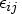is the cross price elasticity for, own price elasticity for i=j, pi is the price on the ith good, and qi is the quantity demanded for the ith good. Price elasticity greater than 1 is called price elastic, and price elasticity smaller than 1 is called price inelastic. A given percentage increase in the price of an elastic good will reduce the quantity demanded for the good by a higher percentage than for an inelastic good.

Income elasticity is defined as the percentage change in quantity demanded with respect to a one percent change in income.where x is total income.

Price elasticities can either be derived from the Marshallian demand equation or the Hicksian demand equation. The Marshallian demand equation is obtained from maximizing utility subject to the budget constraint, while the Hicksian demand equation is derived from solving the dual problem of expenditure minimization at a certain utility level. Elasticities derived from Marshallian demand are called Marshallian or uncompensated elasticities, and elasticities derived from Hicksian demand are called Hicksian or compensated elasticities. Marshallian elasticities can be transformed into Hicksian elasticities through the Slutsky equation:where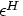represents Hicksian elasticity,represents Marshallian elasticity, wj is the budget share on good j, and ei is the income elasticity for good i. More detailed discussions on the Marshallian and the Hicksian demand relations and the Slutsky equation can be found in many standard economics textbooks; see Nicholson (1992) and Gravelle and Rees (1992).

# Analysis

In this example, you calculate the Marshallian and the Hicksian price elasticities and the income elasticity for the Almost Ideal Demand System (AIDS) model described in the example "Estimating an Almost Ideal Demand System Model." The model is as follows:
where wi is the share associated with the ith good,is the slope coefficient associated with the j th good in the i th share equation, and pj is the price on the j th good. X is the total expenditure on the system of goods, and P is the price index.

The AIDS model implies that the Marshallian price elasticity for good i with respect to good j is
where
Income elasticity is given by
If you are interested in elasticities at a specific point, an ESTIMATE statement can be used in the MODEL procedure to obtain estimates and standard errors of the elasticity at that point. For example, if you want to calculate own price elasticity for beef, and you know both the budget share for beef, say, 0.5, and ln(X/P), say, 9.0, then you can use an ESTIMATE statement in the MODEL procedure as follows (see "Estimating an Almost Ideal Demand System Model" for more code in the MODEL statement):

   data aids_;
input year qtr pop b_q p_q c_q t_q b_p p_p c_p t_p cpi pc_exp;

... more datalines ...

;
run;

   /* Full Nonlinear AIDS Model */
proc model data=aids;

w_b = ab + gbb*lpb + gbp*lpp + gbc*lpc + gbt*lpt + bb*(lx-p) + abco1*co1
+ absi1*si1 + ab_t*t ;
w_p = ap + gbp*lpb + gpp*lpp + gpc*lpc + gpt*lpt + bp*(lx-p) + apco1*co1
+ apsi1*si1 + ap_t*t ;
w_c = ac + gbc*lpb + gpc*lpp + gcc*lpc + gct*lpt + bc*(lx-p) + acco1*co1
+ acsi1*si1 + ac_t*t ;

fit w_b w_p w_c / itsur nestit outs=rest outest=fin2 converge = .00001
maxit = 1000 ;
parms ab bb gbb gbp gbc gbt abco1 absi1 ab_t
ap bp     gpp gpc gpt apco1 apsi1 ap_t
ac bc         gcc gct acco1 acsi1 ac_t
at                gtt ;
estimate 'elasticity beef' (gbb - bb*(.5 - bb*9.0))/.5 - 1;

run;
quit;


This will yield the estimate for the elasticity when the budget share for beef is 0.5, and ln(X/P) = 9.0. The output is shown below.

The MODEL Procedure

Nonlinear ITSUR Estimates
Term Estimate Approx Std Err t Value Approx
Pr > |t|
Label
elasticity beef -0.93823 0.0355 -26.40 <.0001 (gbb - bb*(.5 - bb*9.0))/.5 - 1

The estimated own price elasticity for beef suggests that increasing the price for beef by 1% will reduce the demand for beef by 0.94%. Such information will be useful for setting prices. The ESTIMATE statement also provides standard error estimates.

If you are not interested in standard errors on the elasticities, and you want to compute all own price and cross price elasticities for the system, it is more convenient to do it in IML as shown below. Recall that the parameters estimated in the nonlinear AIDS model are in the data set fin2. The variables not needed in the calculation are eliminated in the following data step so it is easier to read the data set into IML.

To calculate elasticities for the nonlinear AIDS model, you first need to read in the estimated parameters from the output data set fin2:


proc iml;
use fin2;
read all var {gbb gbp gbc gbt gpp gpc gpt gcc gct gtt} ;
read all var {bb bp bc ab ap ac at} ;

close fin2;


Note that the elasticities have meanings only at a specific data point. In the current example, you calculate the elasticities at the mean point of the data. The following example illustrates the nonlinear AIDS case.


/* recall meanw contains the means of the variables */
use meanw;

/* read in the mean shares */
read all var {w_b w_p w_c w_t} ;
/* read in the mean price and expenditure */
read all var {bm pm cm tm x } ;

lpb = log(bm);
lpp = log(pm);
lpc = log(cm);
lpt = log(tm);
lx=log(x);

close meanw;


To calculate the elasticity matrix with own price elasticity as diagonal elements and cross price elasticities as off diagonal elements, you can express the parameters in matrix form and use matrix manipulation in the calculation.


/* Budget share vector */
w = w_b//w_p//w_c//w_t;

/* gamma(i,j) matrix */
gij = (gbb||gbp||gbc||gbt)//
(gbp||gpp||gpc||gpt)//
(gbc||gpc||gcc||gct)//
(gbt||gpt||gct||gtt);

/* turkey parameter based on sum-to-one constraint */
bt= 0-bb-bp-bc;

a=ab//ap//ac//at;  /* alpha(i) vector */
b=bb//bp//bc//bt;  /* beta(i) vector  */


You then specify the nonlinear price index as described in the example "Estimating an Almost Ideal Demand System Model":


a0=0;
p = a0 + ab*lpb + ap*lpp + ac*lpc + at*lpt +
.5*(gbb*lpb*lpb + gbp*lpb*lpp + gbc*lpb*lpc + gbt*lpb*lpt +
gbp*lpp*lpb + gpp*lpp*lpp + gpc*lpp*lpc + gpt*lpp*lpt +
gbc*lpc*lpb + gpc*lpc*lpp + gcc*lpc*lpc + gct*lpc*lpt +
gbt*lpt*lpb + gpt*lpt*lpp + gct*lpt*lpc + gtt*lpt*lpt );


Now you calculate each element of the elasticity matrix:


nk=ncol(gij);

mi = -1#I(nk);

ff2 = j(nk,nk,0);   /* Initialize Marshallian elasticity matrix */
fic2 = j(nk,nk,0);  /* Initialize Hicksian elasticity matrix */
fi2 = j(nk,1,0);    /* Income elasticity vector */

/* prepare for plotting the elasticity matrices*/

/* initialize index vectors for the X- and Y-axis */
x = j(nk*nk,1,0);
y = j(nk*nk,1,0);

/* initialize vector to store elasticity matrices */
Helast = j(nk*nk,1,0);
Melast = j(nk*nk,1,0);

i=1;
do i=1 to nk;
fi2[i,1] = 1 + b[i,]/w[i,];
j=1;
do j=1 to nk;
ff2[i,j] = mi[i,j] + (gij[i,j] - b[i,]#(w[j,]-b[j,]#(lx-p)))/w[i,];
fic2[i,j] = ff2[i,j] + w[j,]#fi2[i,];
x[(i-1)*nk+j,1] = i ;
y[(i-1)*nk+j,1] = j ;
Melast[(i-1)*nk+j,1] = ff2[i,j] ;
Helast[(i-1)*nk+j,1] = fic2[i,j] ;
end;
end;

/*create data set for plotting*/
create plotdata var{x y Melast Helast} ;
append;
close plotdata;
run;
quit;


The calculated Marshallian elasticity matrix for the nonlinear AIDS model is given below:

Marshallian Elasticity Matrix
BEEF PORK CHICKEN TURKEY
BEEF -0.944019 -0.018546 -0.108581 0.0234774
PORK -0.026672 -0.851963 -0.111961 -0.041822
CHICKEN -0.164428 -0.098732 -0.195914 -0.122067
TURKEY 0.0180247 -0.477217 -0.590155 -0.572917

The results show that all own price elasticities are negative, and all of the elasticities are less than 1 in absolute value, meaning that all goods are inelastic.

The income elasticities are reported below:

Income Elasticity
BEEF 1.0476685
PORK 1.0324174
CHICKEN 0.5811402
TURKEY 1.6222649

The results show that turkey consumption is the most sensitive to income changes, while chicken consumption is the least sensitive to income changes.

Finally, the Hicksian elasticity matrix is given below:

Hicksian Elasticity Matrix
BEEF PORK CHICKEN TURKEY
BEEF -0.382661 0.2802386 0.0385055 0.0639165
PORK 0.526515 -0.557529 0.0329848 -0.001971
CHICKEN 0.1469568 0.0670036 -0.114325 -0.099635
TURKEY 0.8872617 -0.014564 -0.362398 -0.510299

The own price Hicksian elasticities are also negative for all four goods as expected.

The following statements illustrate plotting the Hicksian elasticity in three dimensions.

   proc g3d data = plotdata;

scatter x*y=Helast

/ grid
shape='pillar'
color=colorval
caxis=blue
rotate=60
size=2.5
yticknum=4
xticknum=4
zticknum=3
zmin=-1
zmax=1;

run;
quit;


The Hicksian elasticity matrix is plotted in the figure shown below. The red bar indicates positive elasticity, while the green bar indicates negative elasticity.Figure 1: Hicksian Elasticity

# Acknowledgment

The SAS program used in this example is based on code provided by Dr. Barry Goodwin.

# References

Gravelle, H., and Rees, R. (1992), Microeconomics, New York: Longman Publishing.

Nicholson, W. (1992), Microeconomic Theory: Basic Principles and Extensions, Fifth Edition, Fort Worth: Dryden Press.

SAS Institute Inc. (1999), SAS/ETS User's Guide, Version 8, Cary, NC: SAS Institute Inc.